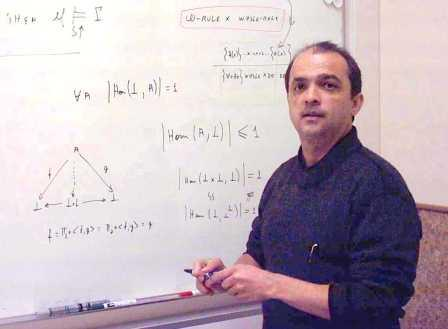How relative can be a mathematical concept ? Introducing the duality "internal versus external" in Category Theory Edward Hermann Haeusler Department of Informatics Pontifical Catholic University of Rio de Janeiro, Brazil Naturality and Universality are key concepts in Mathematics. Category Theory CT was created with the aim of providing means to precisely define naturality in mathematics. Universal properties play a distinguished role in CT. This concept seems to be firstly formalized by Pierre Samuel in 1948 and used in Bourbaki afterwards, although the Bourbaki group did not use Category Theory in their publications. Almost every important categorical construction is defined by means of an universal property: products, sums, limits and co-limits in general, exponentials, subobject classifiers and adjunctions. Regarding this last construction, the well-known "adjoint situations arise everywhere'' shows how worth Naturality and Universality are in CT. Usually the mathematical discourse is ontologically based on parts of Set Theory. The aim of this tutorial is twofold. One goal is to briefly show how the use of universal properties can induce natural equivalences and how both provide interesting logical constructions that can be done inside a class of categories defining what is known as internal logic. The class of categories that having a rich enough internal logical can serve as alternative ontological basis for the mathematical discourse. These categories are known as Topoi. The second goal of this tutorial is to show how an apparently intuitive mathematical concepts, the concept of finiteness, may not be equivalent under equivalent definitions known from Set Theory. A further discussion on the distinction between external and internal logic on categories follows through some examples. The use of Category Theory to provide semantics for logical systems is lastly discussed as appear.Bibliography Goldblatt, R. Topoi: A Categorical Analysis of Logic, North-Holland, 1984. Johnstone, P. Sketches of an Elephant: A Topos Theory Compendium, section D5, Aspects of finiteness. Oxford University Press, 2002. Wolter, U., Martini, A., Haeusler, E.H. "Towards a Uniform Presentation of Logical Systems by Indexed Categories and Adjoint Situations". in Journal of Logic and Computation, to appear 2013, doi:10.1093/logcom/exs038 Spinola, A.I.A and Haeusler, E.H. "Anticipatory Topoi", in AIP Proceedings, 627, pp 543--552. doi:http://dx.doi.org/10.1063/1.1503730.
�>